• 极限学习机matlab代码，极限学习机matlab代码，极限学习机matlab代码极限学习机matlab代码，，极限学习机matlab代码，极限学习机matlab代码
• 伴随深度学习的热潮，极限学习机也向着深度的方向实现，本文浅析如何实现深度极限学习机，并附上MATLAB代码
• 关于基本极限学习机的全部MATLAB代码，全部都是从官网下载，其中还有几个实例可供参考。
• 这是一个在MATLAB上面可以运行的极限学习机算法实例，文件中，包含了多个极限学习机样例，ELM说白了就是另一种神经网络，其作用相似，效果又不同，比如其离散型更强等，建议直接修改接口，方便调试
• 极限学习机MATLAB代码，包括分类和回归的，还有对比实验
• 加权极限学习机 针对数据集中存在的样本不均衡情况所提出的 ELM 改进算法
• 极限学习机matlab代码，可以直接运行，可解决回归和分类问题，且是多分类问题ELM
• 对于入门的ELM学习者，这个代码比较好的对于ELM进行了总结，更改了少量代码
• 代码主要利用MATLAB工具进行MATLAB——极限学习机的回归拟合及分类——比对试验研究的仿真
• 极限学习机(ELM)源代码，黄广斌教授源代码，希望大家一起学习
• PSO-ELM粒子群优化的极限学习机，通过调节参数拟合效果很好，便于大家使用，为学者和科研人员提供基础和思路回归预测
• BP神经网络、ELM极限学习机、SVM支持向量机MATLAB代码，以及测试数据，用于回归预测，相关细节可以在下方评论，看到时一一解答。
• 内含近红外光谱数据，可进行归回和判别分析，数据采集规范有效，代码可顺利一键运行。欢迎下载，共同谈论交流
• 极限学习机(Extreme Learning Machine, ELM) 极限学习机网络结构和BP网络结构类似。
极限学习机(Extreme Learning Machine, ELM)极限学习机网络结构和BP网络结构类似。
和BP神经网络不同点：

极限学习机输入层到隐含层的权重W是可以随意指定的。BP神经网络W层需要在学习时进行调整，而极限学习机不需要调整。由于不需要调整，大大加快了速度。
极限学习机的隐含层到输出层的权重B也不需要迭代调整，而是通过解方程组的方法求出权重。

原理
前向传播图见下
T为目标输出，g为激活函数把上图简洁化，写成矩阵，H为输入层与隐含层相乘的结果该算法创造者认为如果隐含层神经元个数等于输入样本个数相等，则
HB -T 矩阵范数为0,0误差。如果隐含层的神经元个数小于待学习的样本个数，模型可以以一定精度学习，即模型的误差小于一定的值权重学习流程

第一步：随机产生输入层到隐含层之间的权重值W和阈值（偏置）b
第二步：计算隐含层的输出矩阵H
第三步：求解隐含层和输出层之间权重B，因为HB-T的矩阵范数为0，则求解公式如下因为可能存在H的逆矩阵不存在的情况，导致最后B没有解，当时作者提出这个算法的时候给出的解决方法是求伪逆矩阵。
现在的解决方法，如图在H后面加上一个小块矩阵，使可逆。
则权重解为极限学习机相比于BP神经网络，SVM有什么特征

易用性。除了预定义的网络架构外，不需要手动调优任何参数
更快的学习速度。大多数训练可以在毫秒、秒和分钟内完成
更高的泛化性能。在大多数情况下，该算法比BP算法具有更好的泛化性能与SVM相似或优于SVM的泛化性能。
适用于几乎所有的非线性激活函数。几乎所有分段连续(包括不连续)微分、非微分函数)可作为激活函数使用。BP神经网络要求激活函数可导可微分。
适合于完全复杂的激活函数。完全复杂函数也可以作为激活函数使用ELM。

极限学习机( ELM)仿真
重点函数

nargin：n arg in：自动计算出方法输入了几个参数
error：给出错误信息
pinv：求伪逆矩阵
sin / hardlim：涉及到激活函数
elmtrain 自己写的函数，用于ELM训练，记住：每一列代表一个样本

elmtrain.m
function [IW,B,LW,TF,TYPE] = elmtrain(P,T,N,TF,TYPE)
% ELMTRAIN Create and Train a Extreme Learning Machine
% Syntax
% [IW,B,LW,TF,TYPE] = elmtrain(P,T,N,TF,TYPE)
% Description
% Input
% P   - Input Matrix of Training Set  (R*Q)
% T   - Output Matrix of Training Set (S*Q)
% N   - Number of Hidden Neurons (default = Q)
% TF  - Transfer Function:
%       'sig' for Sigmoidal function (default)
%       'sin' for Sine function
%       'hardlim' for Hardlim function
% TYPE - Regression (0,default) or Classification (1)
% Output
% IW  - Input Weight Matrix (N*R)
% B   - Bias Matrix  (N*1)
% LW  - Layer Weight Matrix (N*S)
% Example
% Regression:
% [IW,B,LW,TF,TYPE] = elmtrain(P,T,20,'sig',0)
% Y = elmtrain(P,IW,B,LW,TF,TYPE)
% Classification
% [IW,B,LW,TF,TYPE] = elmtrain(P,T,20,'sig',1)
% Y = elmtrain(P,IW,B,LW,TF,TYPE)
% See also ELMPREDICT
% Yu Lei,11-7-2010
% Copyright www.matlabsky.com
% $Revision:1.0$
if nargin < 2
error('ELM:Arguments','Not enough input arguments.');
end
if nargin < 3
N = size(P,2);
end
if nargin < 4
TF = 'sig';
end
if nargin < 5
TYPE = 0;
end
if size(P,2) ~= size(T,2)
error('ELM:Arguments','The columns of P and T must be same.');
end
[R,Q] = size(P);
if TYPE  == 1
T  = ind2vec(T);
end
[S,Q] = size(T);
% Randomly Generate the Input Weight Matrix
IW = rand(N,R) * 2 - 1;
% Randomly Generate the Bias Matrix
B = rand(N,1);
BiasMatrix = repmat(B,1,Q);
% Calculate the Layer Output Matrix H
tempH = IW * P + BiasMatrix;
switch TF
case 'sig'
H = 1 ./ (1 + exp(-tempH));
case 'sin'
H = sin(tempH);
case 'hardlim'
H = hardlim(tempH);
end
% Calculate the Output Weight Matrix
LW = pinv(H') * T';


elmpredict.m
function Y = elmpredict(P,IW,B,LW,TF,TYPE)
% ELMPREDICT Simulate a Extreme Learning Machine
% Syntax
% Y = elmtrain(P,IW,B,LW,TF,TYPE)
% Description
% Input
% P   - Input Matrix of Training Set  (R*Q)
% IW  - Input Weight Matrix (N*R)
% B   - Bias Matrix  (N*1)
% LW  - Layer Weight Matrix (N*S)
% TF  - Transfer Function:
%       'sig' for Sigmoidal function (default)
%       'sin' for Sine function
%       'hardlim' for Hardlim function
% TYPE - Regression (0,default) or Classification (1)
% Output
% Y   - Simulate Output Matrix (S*Q)
% Example
% Regression:
% [IW,B,LW,TF,TYPE] = elmtrain(P,T,20,'sig',0)
% Y = elmtrain(P,IW,B,LW,TF,TYPE)
% Classification
% [IW,B,LW,TF,TYPE] = elmtrain(P,T,20,'sig',1)
% Y = elmtrain(P,IW,B,LW,TF,TYPE)
% See also ELMTRAIN
% Yu Lei,11-7-2010
% Copyright www.matlabsky.com
% $Revision:1.0$
if nargin < 6
error('ELM:Arguments','Not enough input arguments.');
end
% Calculate the Layer Output Matrix H
Q = size(P,2);
BiasMatrix = repmat(B,1,Q);
tempH = IW * P + BiasMatrix;
switch TF
case 'sig'
H = 1 ./ (1 + exp(-tempH));
case 'sin'
H = sin(tempH);
case 'hardlim'
H = hardlim(tempH);
end
% Calculate the Simulate Output
Y = (H' * LW)';
if TYPE == 1
temp_Y = zeros(size(Y));
for i = 1:size(Y,2)
[~,index] = max(Y(:,i));
temp_Y(index,i) = 1;
end
Y = vec2ind(temp_Y);
end


分类
鸢尾花侯种类识别
这个数据集网上很多，就不放啦
main_iris.m
%% I. 清空环境变量
clear all
clc

%% II. 训练集/测试集产生
%%
% 1. 导入数据
load iris_data.mat

%%
% 2. 随机产生训练集和测试集
P_train = [];
T_train = [];
P_test = [];
T_test = [];
for i = 1:3
temp_input = features((i-1)*50+1:i*50,:);
temp_output = classes((i-1)*50+1:i*50,:);
n = randperm(50);
% 训练集——120个样本
P_train = [P_train temp_input(n(1:40),:)'];
T_train = [T_train temp_output(n(1:40),:)'];
% 测试集——30个样本
P_test = [P_test temp_input(n(41:50),:)'];
T_test = [T_test temp_output(n(41:50),:)'];
end

%% III. ELM创建/训练
[IW,B,LW,TF,TYPE] = elmtrain(P_train,T_train,20,'sig',1);

%% IV. ELM仿真测试
T_sim_1 = elmpredict(P_train,IW,B,LW,TF,TYPE);
T_sim_2 = elmpredict(P_test,IW,B,LW,TF,TYPE);

%% V. 结果对比
result_1 = [T_train' T_sim_1'];
result_2 = [T_test' T_sim_2'];
%%
% 1. 训练集正确率
k1 = length(find(T_train == T_sim_1));
n1 = length(T_train);
Accuracy_1 = k1 / n1 * 100;
disp(['训练集正确率Accuracy = ' num2str(Accuracy_1) '%(' num2str(k1) '/' num2str(n1) ')'])

%%
% 2. 测试集正确率
k2 = length(find(T_test == T_sim_2));
n2 = length(T_test);
Accuracy_2 = k2 / n2 * 100;
disp(['测试集正确率Accuracy = ' num2str(Accuracy_2) '%(' num2str(k2) '/' num2str(n2) ')'])

%% VI. 绘图
figure(2)
plot(1:30,T_test,'bo',1:30,T_sim_2,'r-*')
grid on
xlabel('测试集样本编号')
ylabel('测试集样本类别')
string = {'测试集预测结果对比(ELM)';['(正确率Accuracy = ' num2str(Accuracy_2) '%)' ]};
title(string)
legend('真实值','ELM预测值')回归
汽油辛烷值预测
%% I. 清空环境变量
clear all
clc

%% II. 训练集/测试集产生
%%
% 1. 导入数据
load spectra_data.mat

%%
% 2. 随机产生训练集和测试集
temp = randperm(size(NIR,1));

% 训练集——50个样本
P_train = NIR(temp(1:50),:)';
T_train = octane(temp(1:50),:)';

% 测试集——10个样本
P_test = NIR(temp(51:end),:)';
T_test = octane(temp(51:end),:)';
N = size(P_test,2);

%% III. 数据归一化
%%
% 1. 训练集
[Pn_train,inputps] = mapminmax(P_train);
Pn_test = mapminmax('apply',P_test,inputps);
%%
% 2. 测试集
[Tn_train,outputps] = mapminmax(T_train);
Tn_test = mapminmax('apply',T_test,outputps);

%% IV. ELM创建/训练
[IW,B,LW,TF,TYPE] = elmtrain(Pn_train,Tn_train,30,'sig',0);

%% V. ELM仿真测试
tn_sim = elmpredict(Pn_test,IW,B,LW,TF,TYPE);
%%
% 1. 反归一化
T_sim = mapminmax('reverse',tn_sim,outputps);

%% VI. 结果对比
result = [T_test' T_sim'];
%%
% 1. 均方误差
E = mse(T_sim - T_test);

%%
% 2. 决定系数
N = length(T_test);
R2=(N*sum(T_sim.*T_test)-sum(T_sim)*sum(T_test))^2/((N*sum((T_sim).^2)-(sum(T_sim))^2)*(N*sum((T_test).^2)-(sum(T_test))^2));

%% VII. 绘图
figure(1)
plot(1:N,T_test,'r-*',1:N,T_sim,'b:o')
grid on
legend('真实值','预测值')
xlabel('样本编号')
ylabel('辛烷值')
string = {'测试集辛烷值含量预测结果对比(ELM)';['(mse = ' num2str(E) ' R^2 = ' num2str(R2) ')']};
title(string)代码和数据百度云
链接：https://pan.baidu.com/s/1o08kfLvUN3n5pYyE9ycfWg
提取码：q9vz
复制这段内容后打开百度网盘手机App，操作更方便哦作者：电气工程的计算机萌新-余登武展开全文神经网络
• 摘要：极限学习机（ELM）是当前...本篇博文尽量通俗易懂地对极限学习机的原理进行详细介绍，之后分析如何用MATLAB实现该算法并对代码进行解释。本文主要内容如下：算法的原理、算法程序实现、点击跳转至全部文件下载页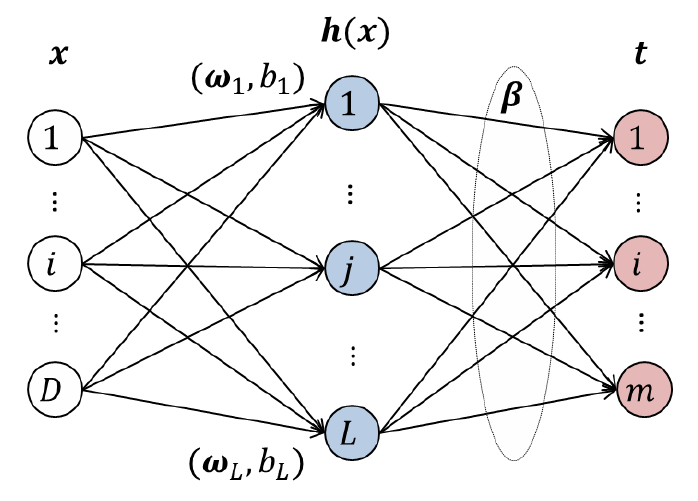摘要：极限学习机（ELM）是当前一类非常热门的机器学习算法，被用来训练单隐层前馈神经网络（SLFN）。本篇博文尽量通俗易懂地对极限学习机的原理进行详细介绍，之后分析如何用MATLAB实现该算法并对代码进行解释。本文主要内容如下：

算法的原理
算法程序实现

点击跳转至全部文件下载页

1. 前言
ELM自2004年南洋理工大学的黄广斌教授提出相关概念以来一直争议不断，但每年相关论文层出不穷，在过去的十年里其理论和应用被广泛研究。如果您想深入学习和了解ELM的原理，博主建议可在ScienceDirect的数据库中检索ELM相关论文，里面有众多优质论文其理解和表述将帮助你更准确了解ELM的内在原理。
博主对于极限学习机（Extreme Learning Machine, ELM）的学习和研究已一年多，目前ELM相关两篇SCI论文已发表。后续转深度学习方向继续研究，这里就ELM作一个简单整理并给出实现代码，也希望对刚接触的朋友能够有所帮助。

2. 算法的原理

极限学习机（ELM）用来训练单隐藏层前馈神经网络（SLFN）与传统的SLFN训练算法不同，极限学习机随机选取输入层权重和隐藏层偏置，输出层权重通过最小化由训练误差项和输出层权重范数的正则项构成的损失函数，依据Moore-Penrose（MP）广义逆矩阵理论计算解析求出。理论研究表明，即使随机生成隐藏层节点，ELM仍保持SLFN的通用逼近能力。在过去的十年里，ELM的理论和应用被广泛研究，从学习效率的角度来看，极限学习机具有训练参数少、学习速度快、泛化能力强的优点。

简单来说，极限学习机（ELM）模型的网络结构与单隐层前馈神经网络（SLFN）一样，只不过在训练阶段不再是传统的神经网络中屡试不爽的基于梯度的算法（后向传播），而采用随机的输入层权值和偏差，对于输出层权重则通过广义逆矩阵理论计算得到。所有网络节点上的权值和偏差得到后极限学习机（ELM）的训练就完成了，这时测试数据过来时利用刚刚求得的输出层权重便可计算出网络输出完成对数据的预测。
ELM的理论推导比较简单，但是需要知道一些线性代数和矩阵论的知识，我会尽量写得简单一些，接下来就一起来推导一下吧。我们假设给定训练集$\left\{\mathrm{x}_{i}, \mathrm{t}_{i} | \mathrm{x}_{i} \in \mathrm{R}^{D}, \mathrm{t}_{i} \in \mathrm{R}^{m}, i=1,2, \ldots, N\right\}$（符号式表达，$\mathrm{x}_{i}$表示第$i$个数据示例，$\mathrm{t}_{i}$表示第$i$个数据示例对应的标记，集合代指所有训练数据），极限学习机的隐藏层节点数为 L，与单隐层前馈神经网络的结构一样，极限学习机的网络结构如下图所示：对于一个神经网络而言，我们完全可以把它看成一个“函数”，单从输入输出看就显得简单许多。很明显上图中，从左往右该神经网络的输入是训练样本集$\mathrm{x}$，中间有个隐藏层，从输入层到隐藏层之间是全连接，记隐藏层的输出为$H(\mathrm{x})$，那么隐藏层输出$H(\mathrm{x})$的计算公式如下。
$H(\mathrm{x})=\left[h_{1}(\mathrm{x}), \ldots, h_{L}(\mathrm{x})\right] \tag{2.1}$    隐藏层的输出是输入乘上对应权重加上偏差，再经过一个非线性函数其所有节点结果求和得到。$\mathrm{H}(\mathrm{x})=\left[h_{1}(\mathrm{x}), \ldots, h_{L}(\mathrm{x})\right]$是ELM非线性映射（隐藏层输出矩阵），$h_{i}(\mathrm{x})$是第$i$个隐藏层节点的输出。隐藏层节点的输出函数不是唯一的，不同的输出函数可以用于不同的隐藏层神经元。通常，在实际应用中，$h_{i}(\mathrm{x})$用如下的表示：
$h_{i}(\mathrm{x})=g\left(\mathrm{w}_{i}, b_{i}, \mathrm{x}\right)=g(\mathrm{w}_{i}\mathrm{x}+\mathrm{b}_{i}), \mathrm{w}_{i} \in \mathrm{R}^{D}, b_{i} \in R\tag{2.2}$    其中$g\left(\mathrm{w}_{i}, b_{i}, \mathrm{x}\right)$（$\mathrm{w}_{i}$和$b_{i}$是隐藏层节点参数）是激活函数，是一个满足ELM通用逼近能力定理的非线性分段连续函数，常用的有Sigmoid函数、Gaussian函数等。如我们可以使用Sigmoid函数，那么式（2.2）中的$g$函数为$g(x)=\frac{1}{1+e^{-x}}=\frac{e^{x}}{e^{x}+1}\tag{2.3}$（将$\mathrm{w}_{i}\mathrm{x}+\mathrm{b}_{i}$代入$x$即可），该函数图像如下图所示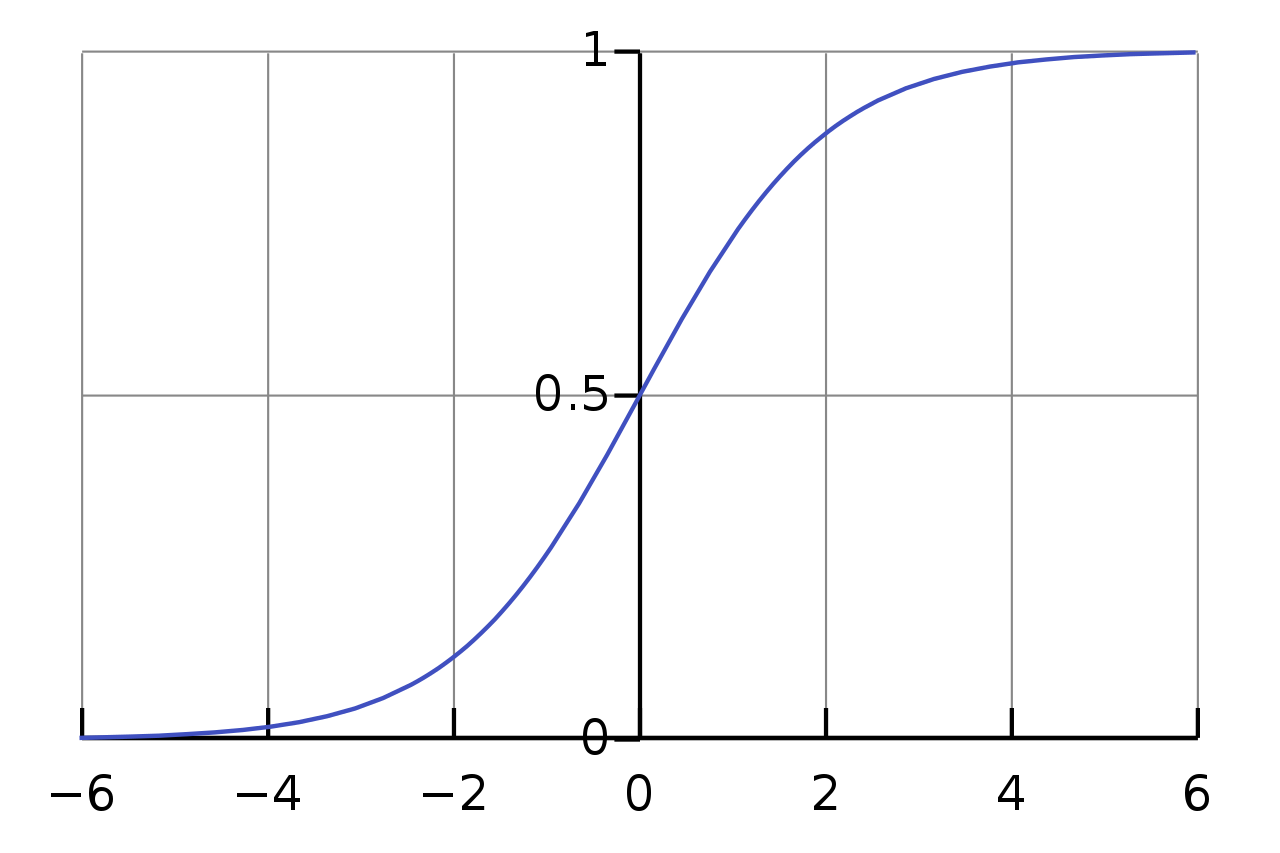经过隐层后进入输出层，根据上面的图示和公式，那么用于“广义”的单隐藏层前馈神经网络ELM的输出是
$f_{L}(\mathrm{x})=\sum_{i=1}^{L} \boldsymbol{\beta}_{i} h_{i}(\mathrm{x})=\mathrm{H}(\mathrm{x}) \boldsymbol{\beta} \tag{2.4}$其中$\boldsymbol{\beta}=\left[\boldsymbol{\beta}_{1}, \ldots, \boldsymbol{\beta}_{L}\right]^{T}$是隐藏层（$L$个节点）与输出层（$m$个节点，$m \geq 1$）之间的输出权重。至此神经网络从输入到输出的操作就是上面公式的计算过程。需要注意的是，目前为止上面公式中的未知量有$\mathrm{w}, \mathrm{b}, \boldsymbol{\beta}$，分别是隐藏层节点上的权值、偏差及输出权值。我们知道神经网络学习（或训练）的过程就是根据训练数据来调整神经元之间的权值以及偏差，而实际上学到的东西则蕴含在连接权值和偏差之中。接下来我们就要用ELM的机理来求解这三个值（ELM训练过程）。
基本上，ELM训练SLFN分为两个主要阶段：（1）随机特征映射（2）线性参数求解。
第一阶段，隐藏层参数随机进行初始化，然后采用一些非线性映射作为激活函数，将输入数据映射到一个新的特征空间（称为ELM特征空间）。简单来说就是ELM隐层节点上的权值和偏差是随机产生的。随机特征映射阶段与许多现有的学习算法（如使用核函数进行特征映射的SVM、深度神经网络中使用限制玻尔兹曼机器（RBM）、用于特征学习的自动编码器/自动解码器）不同。ELM 中的非线性映射函数可以是任何非线性分段连续函数。在ELM中，隐藏层节点参数（$w$ 和$b$）根据任意连续的概率分布随机生成（与训练数据无关），而不是经过训练确定的，从而致使与传统BP神经网络相比在效率方面占很大优势。
经过第一阶段$\mathrm{w}, \mathrm{b}$已随机产生而确定下来，由此可根据公式（2.1）和（2.2）计算出隐藏层输出$H$。在ELM学习的第二阶段，我们只需要求解输出层的权值（$\beta$）。为了得到在训练样本集上具有良好效果的$\beta$，需要保证其训练误差最小，我们可以用$\mathrm{H} \boldsymbol{\beta}$（Hβ是网络的输出，如公式（2.4））与样本标签$T$求最小化平方差作为评价训练误差（目标函数），使得该目标函数最小的解就是最优解。即通过最小化近似平方差的方法对连接隐藏层和输出层的权重（$\beta$）进行求解，目标函数如下：
$\min \|\mathrm{H} \boldsymbol{\beta}-\mathrm{T}\|^{2} , \boldsymbol{\beta} \in \mathbf{R}^{L \times m} \tag{2.5}$其中H是隐藏层的输出矩阵，T是训练数据的目标矩阵：
$H=[h(x_1),...,h(x_{N})]^{T}=\begin{bmatrix} h_1(x_1)&...&h_L(x_1) \\ & ... & \\ h_1(x_N) &...&h_L(x_N) \end{bmatrix},T=\begin{bmatrix} t_{1}^{T}\\ ... \\ t_{N}^{T} \end{bmatrix} \tag{2.6}$通过线代和矩阵论的知识可推导得公式（2.5）的最优解为：
$\beta^{*}=\mathrm{H}^{\dagger} \mathrm{T} \tag{2.7}$其中$\mathrm{H}^{\dagger}$为矩阵H的Moore–Penrose广义逆矩阵。
这时问题就转化为求计算矩阵H的Moore–Penrose广义逆矩阵，该问题主要的几种方法有正交投影法、正交化法、迭代法和奇异值分解法（SVD）。当$H^{T}H$为非奇异（可逆）时可使用正交投影法，这时可得计算结果是：
$\mathbf{H}^{\dagger}=\left(\mathbf{H}^{T} \mathbf{H}\right)^{-1} \mathbf{H}^{T}$    然而有时候在应用时会出现$H^{T}H$为奇异的（不可逆）情况，因此正交投影法并不能很好地应用到所有的情况。由于使用了搜索和迭代，正交化方法和迭代方法具有局限性。而奇异值分解（SVD）总是可以用来计算$H$的Moore–Penrose广义逆，因此用于ELM的大多数实现中。具体的求解过程涉及矩阵论的知识，而且在许多编程语言中都已封装了求解广义逆的可调用的函数，限于篇幅这里博主就不多展开了。至此训练部分全部完成，在测试时利用训练得到的结果便可预测结果。
综合上面说的，对于训练单隐层前馈神经网络的极限学习机算法做出总结，算法如下所示：

算法：极限学习机（ELM）
输入：
数据集：$\left\{\mathrm{x}_{i}, \mathrm{t}_{i} | \mathrm{x}_{i} \in \mathrm{R}^{D}, \mathrm{t}_{i} \in \mathrm{R}^{m}, i=1,2, \ldots, N\right\}$
隐层神经元数目：$L$
激活函数：$g( .)$
输出：
输出权重：$\beta$
1.随机产生输入权重$w$和隐层偏差$b$；
2.计算隐藏层输出$\mathrm{H}=\mathrm{g}(\mathrm{x} * \boldsymbol{\omega}+\mathrm{b})$；
3.采用式（2.7）计算输出层权重$\mathrm{\beta}$；

其实以上的理论尚有不足之处，根据Bartlett理论，对于达到较小的训练误差的前馈神经网络，通常其权值范数越小，网络趋向于获得更好的泛化性能。为了进一步提高传统极限学习机的稳定性和泛化能力，可以在上面目标函数中增加权值范数的约束项来重新求解输出权重，因此提出了优化等式约束的极限学习机。除此之外后来还有许多文章在原有极限学习机的理论和算法上进行了改进，这里就不多介绍了有兴趣的可以了解一下。

3. 算法程序实现
数学中的符号语言可谓精简巧妙，其简洁之美不经间引人入胜，同时它的复杂又让人觉得自己知道的还很匮乏。作为工科的我数学基本功仅限于刚考研时的临阵磨枪，如今的研究生阶段接触了最优理论、统计分析和矩阵论的知识但自感尚不能得其万一。上面的算法推导是完成了，但不代表程序就能完整照上面的步骤实现了，毕竟理论和实际总是有差距的。
其实ELM的程序代码早已开放，提供源码下载的网站：黄广斌老师的ELM资源主页，上面已经有了MATLAB、C++、python和Java的版本，使用起来也比较方便。这里博主就其中MATLAB的代码介绍下算法的程序实现，为方便初学者理解源码，我只是在其中的大部分代码中进行了注释，当然为避免个人理解的偏差建议可去官网查看英文源码。
function [TrainingTime, TestingTime, TrainingAccuracy, TestingAccuracy] = ELM(TrainingData_File, TestingData_File, Elm_Type, NumberofHiddenNeurons, ActivationFunction)
%% ELM 算法程序
% 调用方式: [TrainingTime, TestingTime, TrainingAccuracy, TestingAccuracy] = elm(TrainingData_File, TestingData_File, Elm_Type, NumberofHiddenNeurons, ActivationFunction)
%
% 输入:
% TrainingData_File     - 训练数据集文件名
% TestingData_File      - 测试训练集文件名
% Elm_Type              - 任务类型：0 时为回归任务，1 时为分类任务
% NumberofHiddenNeurons - ELM的隐层神经元数目
% ActivationFunction    - 激活函数类型:
%                           'sig' ， Sigmoidal 函数
%                           'sin' ， Sine 函数
%                           'hardlim' ， Hardlim 函数
%                           'tribas' ， Triangular basis 函数
%                           'radbas' ， Radial basis 函数
% 输出:
% TrainingTime          - ELM 训练花费的时间（秒）
% TestingTime           - 测试数据花费的时间（秒）
% TrainingAccuracy      - 训练的准确率（回归任务时为RMSE，分类任务时为分类正确率）
% TestingAccuracy       - 测试的准确率（回归任务时为RMSE，分类任务时为分类正确率）
%
% 调用示例（回归）: [TrainingTime, TestingTime, TrainingAccuracy, TestingAccuracy] = ELM('sinc_train', 'sinc_test', 0, 20, 'sig')
% 调用示例（分类）: [TrainingTime, TestingTime, TrainingAccuracy, TestingAccuracy] = ELM('diabetes_train', 'diabetes_test', 1, 20, 'sig')

%% 数据预处理

% 定义任务类型
REGRESSION=0;
CLASSIFIER=1;

% 载入训练数据集
train_data=load(TrainingData_File);
T=train_data(:,1)';                   % 第一列：分类或回归的期望输出
P=train_data(:,2:size(train_data,2))';% 第二列到最后一列：不同数据的属性
clear train_data;                     % 清除中间变量

% 载入测试数据集
test_data=load(TestingData_File);
TV.T=test_data(:,1)';                  % 第一列：分类或回归的期望输出
TV.P=test_data(:,2:size(test_data,2))';% 第二列到最后一列：不同数据的属性
clear test_data;                       % 清除中间变量

% 获取训练、测试数据情况
NumberofTrainingData=size(P,2);        % 训练数据中分类对象个数
NumberofTestingData=size(TV.P,2);      % 测试数据中分类对象个数
NumberofInputNeurons=size(P,1);        % 神经网络输入个数，训练数据的属性个数

%% 分类任务时的数据编码
if Elm_Type~=REGRESSION
% 分类任务数据预处理
sorted_target=sort(cat(2,T,TV.T),2);% 将训练数据和测试数据的期望输出合并成一行，然后按从小到大排序
label=zeros(1,1);                   %  Find and save in 'label' class label from training and testing data sets
label(1,1)=sorted_target(1,1);      % 存入第一个标签
j=1;
% 遍历所有数据集标签（期望输出）得到数据集的分类数目
for i = 2:(NumberofTrainingData+NumberofTestingData)
if sorted_target(1,i) ~= label(1,j)
j=j+1;
label(1,j) = sorted_target(1,i);
end
end
number_class=j;                    % 统计数据集（训练数据和测试数据）一共有几类
NumberofOutputNeurons=number_class;% 一共有几类，神经网络就有几个输出

% 预定义期望输出矩阵
temp_T=zeros(NumberofOutputNeurons, NumberofTrainingData);
% 遍历所有训练数据的标记，扩充为num_class*NumberofTraingData的矩阵
for i = 1:NumberofTrainingData
for j = 1:number_class
if label(1,j) == T(1,i)
break;
end
end
temp_T(j,i)=1;                %一个矩阵，行是分类，列是对象，如果该对象在此类就置1
end
T=temp_T*2-1;                     % T为处理的期望输出矩阵，每个对象（列）所在的真实类（行）位置为1，其余为-1

% 遍历所有测试数据的标记，扩充为num_class*NumberofTestingData的矩阵
temp_TV_T=zeros(NumberofOutputNeurons, NumberofTestingData);
for i = 1:NumberofTestingData
for j = 1:number_class
if label(1,j) == TV.T(1,i)
break;
end
end
temp_TV_T(j,i)=1;            % 期望输出表示矩阵，行是分类，列是对象，如果该对象在此类就置1
end
TV.T=temp_TV_T*2-1;              % T为处理的期望输出矩阵，每个对象（列）所在的真实类（行）位置为1，其余为-1

end  % Elm_Type

%% 计算隐藏层的输出H
start_time_train=cputime;           % 训练开始计时

% 随机产生输入权值InputWeight (w_i)和隐层偏差biases BiasofHiddenNeurons (b_i)
InputWeight=rand(NumberofHiddenNeurons,NumberofInputNeurons)*2-1; % 输入节点的权重在[-1,1]之间
BiasofHiddenNeurons=rand(NumberofHiddenNeurons,1);                % 连接偏重在[0,1]之间
tempH=InputWeight*P; % 不同对象的属性*权重
clear P; % 释放空间
ind=ones(1,NumberofTrainingData);     % 训练集中分类对象的个数
BiasMatrix=BiasofHiddenNeurons(:,ind);% 扩展BiasMatrix矩阵大小与H匹配
tempH=tempH+BiasMatrix;               % 加上偏差的最终隐层输入

%计算隐藏层输出矩阵
switch lower(ActivationFunction) % 选择激活函数，lower是将字母统一为小写
case {'sig','sigmoid'}
H = 1 ./ (1 + exp(-tempH));% Sigmoid 函数
case {'sin','sine'}
H = sin(tempH);            % Sine 函数
case {'hardlim'}
H = double(hardlim(tempH));% Hardlim 函数
case {'tribas'}
H = tribas(tempH);         % Triangular basis 函数
case {'radbas'}
H = radbas(tempH);         % Radial basis 函数
% 可在此添加更多激活函数
end
clear tempH;% 释放不在需要的变量

%% 计算输出权重 OutputWeight (beta_i)
OutputWeight=pinv(H') * T';   % 无正则化因子的应用，参考2006年 Neurocomputing 期刊上的论文
% OutputWeight=inv(eye(size(H,1))/C+H * H') * H * T';   % faster method 1 ，参考 2012 IEEE TSMC-B 论文
% OutputWeight=(eye(size(H,1))/C+H * H') \ H * T';      % faster method 2 ，refer to 2012 IEEE TSMC-B 论文

end_time_train=cputime;
TrainingTime=end_time_train-start_time_train; % 计算训练ELM时CPU花费的时间

% 计算输出
Y=(H' * OutputWeight)';                       % Y为训练数据输出（列向量）
if Elm_Type == REGRESSION
TrainingAccuracy=sqrt(mse(T - Y));        % 回归问题计算均方误差根
end
clear H;

%% 计算测试数据的输出（预测标签）
start_time_test=cputime;    % 测试计时
tempH_test=InputWeight*TV.P;% 测试的输入
clear TV.P;

ind=ones(1,NumberofTestingData);
BiasMatrix=BiasofHiddenNeurons(:,ind); % 扩展BiasMatrix矩阵大小与H匹配
tempH_test=tempH_test + BiasMatrix;% 加上偏差的最终隐层输入
switch lower(ActivationFunction)
case {'sig','sigmoid'}% Sigmoid 函数
H_test = 1 ./ (1 + exp(-tempH_test));
case {'sin','sine'}   % Sine 函数
H_test = sin(tempH_test);
case {'hardlim'}      % Hardlim 函数
H_test = hardlim(tempH_test);
case {'tribas'}       % Triangular basis 函数
H_test = tribas(tempH_test);
case {'radbas'}       % Radial basis 函数
H_test = radbas(tempH_test);
% 可在此添加更多激活函数
end
TY=(H_test' * OutputWeight)';                       %   TY: 测试数据的输出

end_time_test=cputime;
TestingTime=end_time_test-start_time_test;          % 计算ELM测试集时CPU花费的时间

%% 计算准确率
if Elm_Type == REGRESSION
TestingAccuracy=sqrt(mse(TV.T - TY));           % 回归问题计算均方误差根
end

% 如果是分类问题计算分类的准确率
if Elm_Type == CLASSIFIER
MissClassificationRate_Training=0;
MissClassificationRate_Testing=0;
% 计算训练集上的分类准确率
for i = 1 : size(T, 2)
[x, label_index_expected]=max(T(:,i));
[x, label_index_actual]=max(Y(:,i));
if label_index_actual~=label_index_expected
MissClassificationRate_Training=MissClassificationRate_Training+1;
end
end
% 计算测试集上的分类准确率
TrainingAccuracy=1-MissClassificationRate_Training/size(T,2); % 训练集分类正确率
for i = 1 : size(TV.T, 2)
[x, label_index_expected]=max(TV.T(:,i));
[x, label_index_actual]=max(TY(:,i));
if label_index_actual~=label_index_expected
MissClassificationRate_Testing=MissClassificationRate_Testing+1;
end
end
TestingAccuracy=1-MissClassificationRate_Testing/size(TV.T,2);  % 测试集分类正确率
end

在以上代码中博主已相当详实地进行了注释，需要注意的是程序中默认训练和测试文件中第一列上的数据为样本标记，其余所有列中数据为样本属性，因此在调用该函数时应先保证自己的数据集已经整理为正确的格式，如下面整理好的Iris数据集（第一列为样本标号）。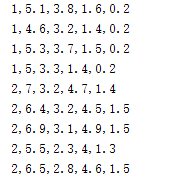至于数据集如何整理可参考博主前面的博文：UCI数据集整理（附论文常用数据集），其中提供了详细的数据集和相关介绍。这里我们写一个测试函数验证以上的ELM函数，对于分类任务我们选取经典的UCI Iris数据集，测试的代码如下：
clear all
clc

dataSet = load('iris.txt');                             % 载入数据集
len_dataSet = size(dataSet,1);                          % 数据集样本数
ind = randperm(len_dataSet);                            % 随机挑选数据
train_set = dataSet(ind(1:round(len_dataSet*0.7)),:);   % 随机的70%数据作为训练集
test_set = dataSet(ind(round(len_dataSet*0.7)+1:end),:);% 随机的30%数据作为测试集

save iris_train.txt -ascii train_set                    % 保存训练集为txt文件
save iris_test.txt -ascii test_set                      % 保存测试集为txt文件

% 调用ELM函数
[TrainingTime, TestingTime, TrainingAccuracy, TestingAccuracy] = ELM('iris_train.txt', 'iris_test.txt', 1, 20, 'sig');

% 输出结果
fprintf('训练集准确率：%g \n',TrainingAccuracy);
fprintf('测试集准确率：%g \n',TestingAccuracy);

运行结果如下：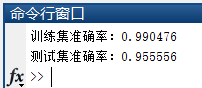对于回归任务可选取Sinc的数据集（该数据已整理分为训练和测试集），测试的代码如下：
clear all
clc

% 调用ELM函数
[TrainingTime,TestingTime,TrainingAccuracy,TestingAccuracy] = ELM('sinc_train','sinc_test',0,20,'sig');

% 输出结果
fprintf('训练集MSE：%g \n',TrainingAccuracy);
fprintf('测试集MSE：%g \n',TestingAccuracy);

运行结果如下：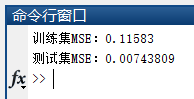【下载链接】
若您想获得博文中涉及的实现完整全部程序文件（包括数据集，m, txt文件等，如下图），这里已打包上传至博主的CSDN下载资源中，下载后运行run_ELM1.m或run_ELM2.m文件即可运行。文件下载链接如下：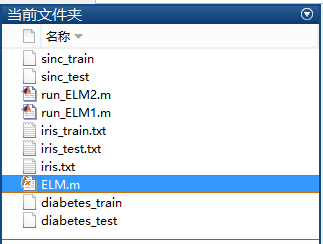下载链接1：博文中涉及的完整程序文件（CSDN下载）
下载链接2：博文中涉及的完整程序文件（面包多下载）
【公众号获取】
本人微信公众号已创建，扫描以下二维码并关注公众号“AI技术研究与分享”，后台回复“EF20190607”即可获取全部资源文件。5. 结束语
由于博主能力有限，博文中提及的方法与代码即使经过测试，也难免会有疏漏之处。希望您能热心指出其中的错误，以便下次修改时能以一个更完美更严谨的样子，呈现在大家面前。同时如果有更好的实现方法也请您不吝赐教。
大家的点赞和关注是博主最大的动力，博主所有博文中的代码文件都可分享给您，如果您想要获取博文中的完整代码文件，可通过C币或积分下载，没有C币或积分的朋友可在关注、点赞博文后提供邮箱，我会在第一时间发送给您。博主后面会有更多的分享，敬请关注哦！


展开全文ELM 机器学习 神经网络
• ELM的程序代码早已开放，提供源码下载的网站：黄广斌老师的ELM资源主页 黄广斌老师的ELM资源主页.，上面已经有了MATLAB、C++、python和Java的版本，使用起来也比较方便。 本文对其代码进行讲解注释。 function ...
ELM的程序代码早已开放，提供源码下载的网站：黄广斌老师的ELM资源主页 黄广斌老师的ELM资源主页.，上面已经有了MATLAB、C++、python和Java的版本，使用起来也比较方便。
本文对其代码进行讲解注释，数据集和原始代码请到上述链接自取。
function [TrainingTime, TestingTime, TrainingAccuracy, TestingAccuracy] = elm(TrainingData_File, TestingData_File, Elm_Type, NumberofHiddenNeurons, ActivationFunction)

%%%%%%%%%%% 定义标签，区分回归问题和分类问题
REGRESSION=0;
CLASSIFIER=1;

%%%%%%%%%%% 载入训练集
train_data=load(TrainingData_File);
T=train_data(:,1)';%训练集标签
P=train_data(:,2:size(train_data,2))';%训练集输入
clear train_data;  %释放内存

%%%%%%%%%%% 载入测试集
test_data=load(TestingData_File);
TV.T=test_data(:,1)';
TV.P=test_data(:,2:size(test_data,2))';
clear test_data;                                    %   Release raw testing data array

NumberofTrainingData=size(P,2);  %训练数据个数
NumberofTestingData=size(TV.P,2); %测试数据个数
NumberofInputNeurons=size(P,1); %输入个数

if Elm_Type~=REGRESSION  %分类问题
%%%%%%%%%%%% Preprocessing the data of classification
sorted_target=sort(cat(2,T,TV.T),2); %将所有标签降序排列
label=zeros(1,1);                     %   Find and save in 'label' class label from training and testing data sets
label(1,1)=sorted_target(1,1);%最大的种类的值
j=1;
for i = 2:(NumberofTrainingData+NumberofTestingData)
if sorted_target(1,i) ~= label(1,j)
j=j+1;  %存储不同种类标签的个数
label(1,j) = sorted_target(1,i);%存储不同种类标签的值
end
end
number_class=j; %不同种类标签的个数
NumberofOutputNeurons=number_class; %输出层神经元个数等于不同种类标签的个数

%%%%%%%%%% Processing the targets of training 处理训练数据的标签
temp_T=zeros(NumberofOutputNeurons, NumberofTrainingData);
for i = 1:NumberofTrainingData
for j = 1:number_class
if label(1,j) == T(1,i)
break;
end
end
temp_T(j,i)=1; %将标签“1”处理为“100000...”
end
T=temp_T*2-1; %将标签“1  0"处理为“1  -1”

%%%%%%%%%% Processing the targets of testing  处理测试数据的标签，同上
temp_TV_T=zeros(NumberofOutputNeurons, NumberofTestingData);
for i = 1:NumberofTestingData
for j = 1:number_class
if label(1,j) == TV.T(1,i)
break;
end
end
temp_TV_T(j,i)=1;
end
TV.T=temp_TV_T*2-1;

end                                                 %   end if of Elm_Type

%%%%%%%%%%% Calculate weights & biases
start_time_train=cputime; %记录开始训练的时间

%%%%%%%%%%% Random generate input weights InputWeight (w_i) and biases BiasofHiddenNeurons (b_i) of hidden neurons
InputWeight=rand(NumberofHiddenNeurons,NumberofInputNeurons)*2-1; %随机输出层权重（-1，1）
BiasofHiddenNeurons=rand(NumberofHiddenNeurons,1); %随机输出层偏置  （0，1）
tempH=InputWeight*P; %计算wx
clear P;                                            %   Release input of training data
ind=ones(1,NumberofTrainingData);
BiasMatrix=BiasofHiddenNeurons(:,ind);              %   Extend the bias matrix BiasofHiddenNeurons to match the demention of H
tempH=tempH+BiasMatrix; %计算隐含层输入wx+b

%%%%%%%%%%% Calculate hidden neuron output matrix H
switch lower(ActivationFunction) %激活函数 、计算隐含层输出H
case {'sig','sigmoid'}
%%%%%%%% Sigmoid
H = 1 ./ (1 + exp(-tempH));
case {'sin','sine'}
%%%%%%%% Sine
H = sin(tempH);
case {'hardlim'}
%%%%%%%% Hard Limit
H = double(hardlim(tempH));
case {'tribas'}
%%%%%%%% Triangular basis function
H = tribas(tempH);
case {'radbas'}
%%%%%%%% Radial basis function
H = radbas(tempH);
%%%%%%%% More activation functions can be added here
end
clear tempH;                                        %   Release the temparary array for calculation of hidden neuron output matrix H

%%%%%%%%%%% Calculate output weights OutputWeight (beta_i)
OutputWeight=pinv(H') * T';  %伪逆，最小二乘计算输出矩阵      % 没有正则化因子的实现 //refer to 2006 Neurocomputing paper

%OutputWeight=inv(eye(size(H,1))/C+H * H') * H * T';   % faster method 1 //refer to 2012 IEEE TSMC-B paper
%implementation; one can set regularizaiton factor C properly in classification applications
%OutputWeight=(eye(size(H,1))/C+H * H') \ H * T';      % faster method 2 //refer to 2012 IEEE TSMC-B paper
%implementation; one can set regularizaiton factor C properly in classification applications

end_time_train=cputime; %记录结束时间
TrainingTime=end_time_train-start_time_train        %   Calculate CPU time (seconds) spent for training ELM

%%%%%%%%%%% Calculate the training accuracy
Y=(H' * OutputWeight)';                             %   Y: the actual output of the training data
if Elm_Type == REGRESSION
TrainingAccuracy=sqrt(mse(T - Y))               %   Calculate training accuracy (RMSE) for regression case
end
clear H;

%%%%%%%%%%% Calculate the output of testing input
start_time_test=cputime;
tempH_test=InputWeight*TV.P;
clear TV.P;             %   Release input of testing data
ind=ones(1,NumberofTestingData);
BiasMatrix=BiasofHiddenNeurons(:,ind);              %   Extend the bias matrix BiasofHiddenNeurons to match the demention of H
tempH_test=tempH_test + BiasMatrix;
switch lower(ActivationFunction)
case {'sig','sigmoid'}
%%%%%%%% Sigmoid
H_test = 1 ./ (1 + exp(-tempH_test));
case {'sin','sine'}
%%%%%%%% Sine
H_test = sin(tempH_test);
case {'hardlim'}
%%%%%%%% Hard Limit
H_test = hardlim(tempH_test);
case {'tribas'}
%%%%%%%% Triangular basis function
H_test = tribas(tempH_test);
case {'radbas'}
%%%%%%%% Radial basis function
H_test = radbas(tempH_test);
%%%%%%%% More activation functions can be added here
end
TY=(H_test' * OutputWeight)';                       %   TY: the actual output of the testing data
end_time_test=cputime;
TestingTime=end_time_test-start_time_test           %   Calculate CPU time (seconds) spent by ELM predicting the whole testing data

if Elm_Type == REGRESSION
TestingAccuracy=sqrt(mse(TV.T - TY))            %   Calculate testing accuracy (RMSE) for regression case
end

if Elm_Type == CLASSIFIER
%%%%%%%%%% Calculate training & testing classification accuracy
MissClassificationRate_Training=0;
MissClassificationRate_Testing=0;

for i = 1 : size(T, 2)
[x, label_index_expected]=max(T(:,i));
[x, label_index_actual]=max(Y(:,i));
if label_index_actual~=label_index_expected
MissClassificationRate_Training=MissClassificationRate_Training+1;
end
end
TrainingAccuracy=1-MissClassificationRate_Training/size(T,2)
for i = 1 : size(TV.T, 2)
[x, label_index_expected]=max(TV.T(:,i));
[x, label_index_actual]=max(TY(:,i));
if label_index_actual~=label_index_expected
MissClassificationRate_Testing=MissClassificationRate_Testing+1;
end
end
TestingAccuracy=1-MissClassificationRate_Testing/size(TV.T,2)
end



展开全文人工智能
• 极限学习机代码，内含数据train data、test data 实例等，可供大家学习，matlab版，亲测可用。
• 极限学习机matlab代码，跑了许多次，表示可以用，之前输出是一维，现在改成了四维。
• 极限学习机ELM的matlab代码，可用于解决分类问题，回归问题
• 基于麻雀搜索算法的极限学习机(ELM)分类算法 文章目录基于麻雀搜索算法的极限学习机(ELM)分类算法1.极限学习机原理概述2.ELM学习算法3.分类问题4.基于麻雀搜索算法优化的ELM5.测试结果6.参考文献7.Matlab代码 摘要：...
基于麻雀搜索算法的极限学习机(ELM)分类算法
文章目录基于麻雀搜索算法的极限学习机(ELM)分类算法1.极限学习机原理概述2.ELM学习算法3.分类问题4.基于麻雀搜索算法优化的ELM5.测试结果6.参考文献7.Matlab代码
摘要：本文利用麻雀搜索算法对极限学习机进行优化，并用于分类问题
1.极限学习机原理概述
典型的单隐含层前馈神经网络结构如图1 所示，由输入层、隐含层和输出层组成，输 入层与隐含层、隐含层与输出层神经元间全连接。其中，输入层有 n 个神经元，对应 n 个输入变量， 隐含层有 l个神经元；输出层有 m 个神经元 ，对应 m 个输出变量 。 为不失一般性，设输 入层与隐含层间的连接权值 w 为:
$w =\left[\begin{matrix}w_{11}&w_{12}&...&w_{1,n}\\ w_{21}&w_{22}&...&w_{2n}\\ ...\\ w_{l1}&w_{l2}&...&w_{ln} \end{matrix}\right]\tag{1}$
其中，$w_n$表示输入层第$i$个神经元与隐含层第$j$个神经元间的连接权值。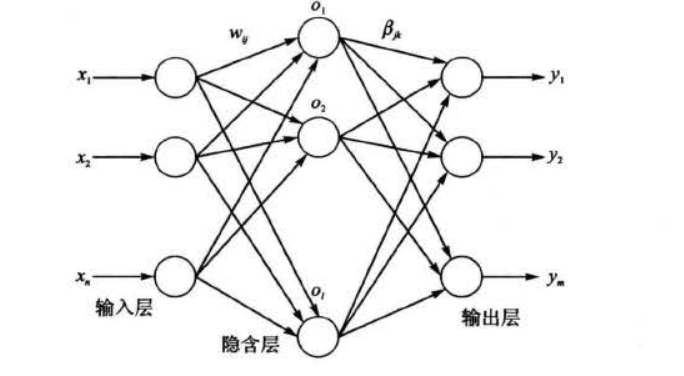设隐含层与输出层间的连接权值 ， 为$\beta$:
$\beta =\left[\begin{matrix} \beta_{11}&\beta_{12}&...&\beta_{1m}\\ \beta_{21}&\beta_{22}&...&\beta_{2m}\\ ...\\ \beta_{l1}&\beta_{l2}&...&\beta_{lm} \end{matrix}\right] \tag{2}$
其中，自$\beta_{jk}$表示隐含层第 j 个神经元与输出层第 k个神经元间的连接权值。
设隐含层神经元的阈值值 b 为：
$b =\left[\begin{matrix}b_1\\ b_2\\ ...\\ b_l \end{matrix}\right]\tag{3}$
设具有 Q 个样本的训练集输入矩阵 X 和输出矩阵 Y 分别为
$X =\left[\begin{matrix}x_{11}&x_{12}&...&x_{1Q}\\ x_{21}&x_{22}&...&x_{2Q}\\ ...\\ x_{n1}&x_{n2}&...&x_{nQ} \end{matrix}\right]\tag{4}$
$Y =\left[\begin{matrix}y_{11}&y_{12}&...&y_{1Q}\\ y_{21}&y_{22}&...&y_{2Q}\\ ...\\ y_{m1}&y_{m2}&...&y_{mQ} \end{matrix}\right]\tag{5}$
设隐含层神经元的激活函数为 g(x)，则由图1 可得， 网络的输出 T 为:
$T = [t_1,..,t_Q]_{m*Q},t_j = [t_{1j},...,t_{mj}]^T =\left[\begin{matrix}\sum_{i=1}^t\beta_{i1}g(w_ix_j + b_i)\\ \sum_{i=1}^t\beta_{i2}g(w_ix_j + b_i)\\ ...\\ \sum_{i=1}^t\beta_{im}g(w_ix_j + b_i) \end{matrix}\right]_{m*1},(j=1,2,...,Q)\tag{6}$
式(6)可表示为：
$H\beta = T’ \tag{7}$
其中， T’为矩阵 T 的转置； H 称为神经网络的隐含层输出矩阵 ， 具体形式如下 ：
$H(w_1,...,w_i,b_1,...,b_l,x_1,...,x_Q) =\left[\begin{matrix} g(w_1*x_1 + b_1)&g(w_2*x_1 + b_2)&...&g(w_l*x_1 + b_l)\\ g(w_1*x_2 + b_1)&g(w_2*x_2 + b_2)&...&g(w_l*x_2 + b_l)\\ ...\\ g(w_1*x_Q + b_1)&g(w_2*x_Q + b_2)&...&g(w_l*x_Q + b_l) \end{matrix}\right]_{Q*l}$
2.ELM学习算法
由前文分析可知，ELM在训练之前可以随机产生 w 和 b ， 只需确定隐含层神经元个数及隐含层和神经元的激活函数（无限可微） ， 即可计算出$\beta$ 。具体地， ELM 的学习算法主要有以下几个步骤：
（1）确定隐含层神经元个数，随机设定输入层与隐含层间的连接权值 w 和隐含层神经元的偏置 b ;
（2） 选择一个无限可微的函数作为隐含层神经元的激活函数，进而计算隐含层输出矩 阵 H ;
（3）计算输出层权值：$\beta = H^+T'$
值得一提的是，相关研究结果表明，在 ELM 中不仅许多非线性激活函数都可以使用（如 S 型函数、正弦函数和复合函数等)，还可以使用不可微函数，甚至可以使用不连续的函数作为激 活函数。
3.分类问题
本文对乳腺肿瘤数据进行分类。采用随机法产生训练集和测试集，其中训练集包含 500 个样本，测试集包含 69 个样本 。
4.基于麻雀搜索算法优化的ELM
麻雀搜索算法的具体原理参考博客：https://blog.csdn.net/u011835903/article/details/108830958。
由前文可知，ELM的初始权值和阈值都是随机产生。每次产生的初始权值和阈值具有满目性。本文利用麻雀搜索算法对初始权值和阈值进行优化。适应度函数设计为训练集的错误率与测试集的错误率的和，以期望使训练得到的网络在测试集和训练集上均有较好的结果:
$fitness = argmin(TrainErrorRate + TestErrorRate)。$
5.测试结果
麻雀算法相关参数如下：
%训练数据相关尺寸
R = size(Pn_train,1);
S = size(Tn_train,1);
N = 20;%隐含层个数
%% 定义麻雀优化参数
pop=20; %种群数量
Max_iteration=50; %  设定最大迭代次数
dim = N*R + N*S;%维度，即权值与阈值的个数
lb = [-1.*ones(1,N*R),zeros(1,N*S)];%下边界
ub = [ones(1,N*R),ones(1,N*S)];%上边界

将经过麻雀优化后的SSA-ELM与基础ELM进行对比。
预测结果如下图
麻雀收敛曲线如下：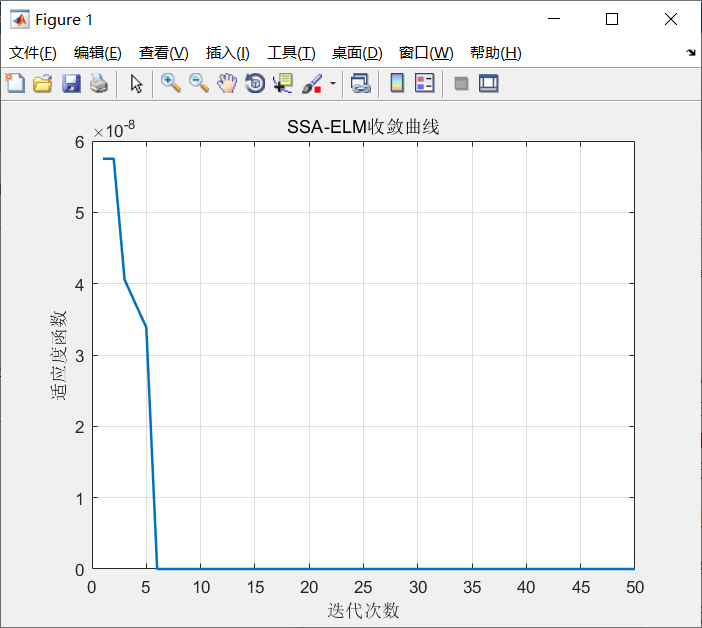数据结果如下：
麻雀优化ELM结果展示：----------------
训练集正确率Accuracy = 99%(495/500)
测试集正确率Accuracy = 95.6522%(66/69)
病例总数：569  良性：357  恶性：212
训练集病例总数：500  良性：318  恶性：182
测试集病例总数：69  良性：39  恶性：30
良性乳腺肿瘤确诊：39  误诊：0  确诊率p1=100%
恶性乳腺肿瘤确诊：27  误诊：3  确诊率p2=90%
传统ELM结果展示：----------------
训练集正确率Accuracy = 92.8%(464/500)
测试集正确率Accuracy = 84.058%(58/69)
病例总数：569  良性：357  恶性：212
训练集病例总数：500  良性：318  恶性：182
测试集病例总数：69  良性：39  恶性：30
良性乳腺肿瘤确诊：36  误诊：3  确诊率p1=92.3077%
恶性乳腺肿瘤确诊：22  误诊：8  确诊率p2=73.3333%
从上述数据可以看出，SSA-ELM训练得到的网络，无论是在测试集和训练集上的正确率均高于基础ELM训练得到的网络。SSA-ELM具有较好的性能。
6.参考文献
书籍《MATLAB神经网络43个案例分析》
7.Matlab代码
https://mianbaoduo.com/o/bread/mbd-YZWXm55r


展开全文神经网络 算法
• 极限学习机Matlab代码，有训练集函数，预测函数，和一个适用范例，全方位指导elm学习算法。
• ## ELM 极限学习机

热门讨论 2013-07-24 21:10:52
极限学习机matlab源码及对应几篇重要文献，对学习人工智能的朋友很有价值！里面有matlab测试源代码和测试数据，非常好用，可用于分类和回归，速度很快。我用9030*1569的数据训练和测试12秒即有结果。# matlab代码极限学习机matlab 订阅